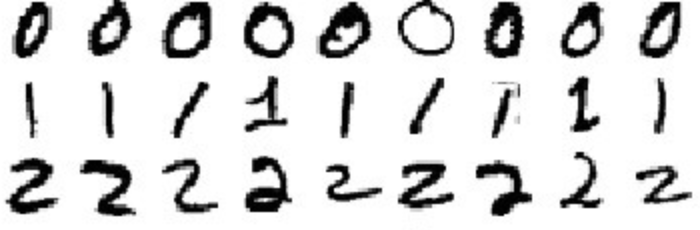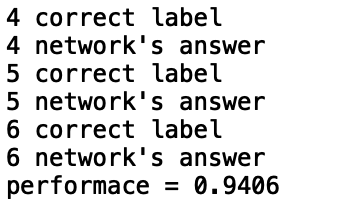# Computer Vision

Brian S. Haney

Introduction

Generally, Artificial Intelligence (“AI”) refers to a machine with the ability to replicate cognitive activities associated with human thought. The goal for many AI researchers is whole brain emulation, which describes machine intelligence copying the computational structure of the human brain. Computer vision, the study of visual data, is one important aspect of achieving this goal. This article will focus on describing the role of convolutional neural networks in computer vision.

Convolutional Neural Networks (CNNs) achieve state-of-the-art performance in computer vision tasks. Generally, deep learning allows machines to learn with architectures inspired by the biological neocortex. Unsurprisingly, CNNs are a deep learning mechanism modeled upon the biological visual cortex. The biological visual cortex is composed of receptive fields made up of cells that are sensitive to small sub-regions of the visual field. In an artificial visual cortex, the response of a neuron to a stimulus in its receptive field is modeled with a mathematical convolutional operation. Convolution is a form of mathematical operation with two matrices: an input matrix and a kernel, or filter. A kernel is a small square matrix that is applied each element of the input matrix.

Generally, a neural network is a function, or transformation of information, operating on input data allowing the abstraction of meaning from the corresponding output. Further, in a CNN Each kernel is convolved across an input matrix and the resulting output is called a feature map. The full output of the layers is obtained by stacking all of the feature maps to create dimensionality. In contrast to some DNNs, the weight coefficients in a CNN are not all connected. Instead, a window is defined over a smaller input space and the units are connected to a small subset of the inputs. In other words, the kernel is centered over a subset of the input matrix and then multiplied for the purpose of feature abstraction. And, the flexibilities of CNNs allow them to be constantly improved with novel architecture design.

Data

The most important part of any neural network is the data. In the two examples in this article, the dataset is the popular MNIST Dataset. The MNIST Database of Handwritten Digits includes a training set of 60,000 examples, and a test set of 10,000 examples.Random samples from MNIST Dataset.

The digits have been size-normalized and centered in a fixed-size image. This dataset is often used for training and introductions to CNNs.

Code

The following examples are CNNs for the MNIST dataset. The first example uses the Python library Numpy. The second example uses Keras, the TensorFlow API.

Example 1

The script begins by importing the required the packages. Numpy is a programming package for scientific computing in Python and the main package in this example. The script also imports scripy.special and matplotlib.pyplot.

```#Import packagesimport numpy#Import scripy.special for the sigmoid functionimport scipy.special#Import library for plotting arraysimport matplotlib.pyplot
```

Next, the script defines a neural network class. The __init__ function initializes the neural network by defining the input, hidden, and output nodes. The ‘self’ parameter assigns the function to the object, an instance of the neuralNetwork class.

```#Neural network class definitionclass neuralNetwork:#initialize the neural networkdef __init__(self, inputnodes, hiddennodes, outputnodes, learningrate):#set number of nodes in each input, hidden, output layerself.inodes = inputnodesself.hnodes = hiddennodesself.onodes = outputnodes#link weight matrices, wih and who#weights inside the arrays are w_i_j, where link is from node i to node j in the next layer#w11 w21 -> w12 w22 etcself.wih = numpy.random.normal(0.0, pow(self.inodes, -0.5), (self.hnodes, self.inodes))self.who = numpy.random.normal(0.0, pow(self.hnodes, -0.5), (self.onodes, self.hnodes))
```
``` #learning rateself.lr = learningrate#activation function is the sigmoid functionself.activation_function = lambda x: scipy.special.expit(x)pass
```

Third, a function is defined for training the neural network. The ‘train’ function includes three parameters ‘self’, ‘inputs_list’, and ‘target_list’ within its definition. Here, the inputs of the function flow forward, where the target reflects the desired answer. Additionally, hidden layers, output layers, and weight updates are defined.

```#Train neural networkdef train(self, inputs_list, targets_list):#Convert inputs list to 2d arrayinputs = numpy.array(inputs_list, ndmin=2).Ttargets = numpy.array(targets_list, ndmin=2).T#calculate signals into hidden layerhidden_inputs = numpy.dot(self.wih, inputs)
```
``` #calculate the signals emerging from hidden layerhidden_outputs = self.activation_function(hidden_inputs)#calculate signals into final output layerfinal_inputs = numpy.dot(self.who, hidden_outputs)#calculate signals emerging from final output layerfinal_outputs = self.activation_function(final_inputs)#output layer error is the (target-actual)output_errors = targets — final_outputs
```
``` #hidden layer error is the output_errors, split by weights, recombined at hidden nodeshidden_errors = numpy.dot(self.who.T, output_errors)#update the weights for the links between the hidden and output layersself.who += self.lr * numpy.dot((output_errors * final_outputs * (1.0 — final_outputs)), numpy.transpose(hidden_outputs))#update the weights for the links between the input and hidden layersself.wih += self.lr * numpy.dot((hidden_errors * hidden_outputs * (1.0 — hidden_outputs)), numpy.transpose(inputs))
```
``` pass
```

Then, a function is defined to query the network. And, the networks signals are calculated.

```#query the neural networkdef query(self, inputs_list):#convert inputs list to 2d arrayinputs = numpy.array(inputs_list, ndmin=2).T
```
```#calculate signals into hidden layerhidden_inputs = numpy.dot(self.wih, inputs)#calculate signals emerging from hidden layerhidden_outputs = self.activation_function(hidden_inputs)#calculate signals into final output layerfinal_inputs = numpy.dot(self.who, hidden_outputs)#calculate the signals emerging from final output layerfinal_outputs = self.activation_function(final_inputs)return final_outputs
```

Fifth, the network’s nodes are defined along with the learning rate. Here, there are 784 input nodes because the MNIST dataset stores values as a 28 x 28 pixel array. And, there are 10 output nodes because there are 10 labels for each of the numbers in the dataset, 0–9.

```#number of input, hidden and output nodesinput_nodes = 784hidden_nodes = 100output_nodes = 10
```
```#learning rate is 0.3learning_rate = 0.3
```

Then, an instance of the network class is created and the training data is loaded. Here, the training data was downloaded from the MNIST website and stored locally in a .csv file.

```#create instance of neural networkn = neuralNetwork(input_nodes, hidden_nodes, output_nodes, learning_rate)
```
```#load the MNIST training data csvfile into a listtraining_data_file = open(“mnist_train.csv”, ‘r’)training_data_list = training_data_file.readlines()training_data_file.close()
```

Next, the neural network is trained through an iterative process. The split method, divides the data by commas.

```#train neural network
```
```#go through all records in the training data setfor record in training_data_list:
```
``` #split the record by the ‘,’ commasall_values = record.split(‘,’)
```
``` #scale and shift the inputsinputs = (numpy.asfarray(all_values[1:]) / 225.0 * 0.99) + 0.01
```
``` #create the target output values(all 0.01, except the desired label which is 0.99)targets = numpy.zeros(output_nodes) + 0.01
```
``` #all_values is the target label for this recordtargets[int(all_values)] = 0.99n.train(inputs, targets)pass
```

Then, the test data is loaded.

```#load the mnist test data csv file into a listtest_data_file = open(“mnist_test.csv”, ‘r’)test_data_list = test_data_file.readlines()test_data_file.close()
```
```#get the first test recordall_values = test_data_list.split(‘,’)
```
```image_array = numpy.asfarray(all_values[1:]).reshape((28,28))
```

The network is then tested and a scorecard displays the results. The scorecard identifies the correct label and the network’s answer.

```#test the neural network#scorecard for how well the network performs, initially empty scorecard = []
```
```#go through all the records in the test data setfor record in test_data_list:
```
``` #split the record by the ‘,’ commasall_values = record.split(‘,’)
```
``` #correct answer is first valuecorrect_label = int(all_values)print(correct_label, “correct label”)
```
``` #scale and shift inputsinputs = (numpy.asfarray(all_values[1:])/ 255.0 * 0.99)+0.01
```
``` #query the networkoutputs = n.query(inputs)
```
``` # the index of the highest value corresponds to the labellabel = numpy.argmax(outputs)print(label, “network’s answer”)
```
``` #append correct or incorrect to listif (label == correct_label):#network’s answer matches correct answer, add 1 to scorecardscorecard.append(1)else:#network’s answer doesn’t match correct answer, add 0 to scorecard.append(0)passpass
```
```#calculate the performance score, the fraction of correct answersscorecard_array = numpy.asarray(scorecard)print(“performace =”, scorecard_array.sum() / scorecard_array.size)
```

The network’s output looks something like this:Numpy CNN Performance

Here, the CNN written in Numpy predicted the correct number with roughly 94% accuracy.

Example 2

Keras is Google’s high-level TensorFlow API for building and training deep learning models. The API is built on top of TensorFlow and is accessible through the new TensorFlow 2.0 Alpha, which was released in early 2019. TensorFlow 2.0 Alpha includes the Keras API through tf.keras module.

First, the packages are imported and the data is defined.

```from __future__ import absolute_import, division, print_function, unicode_literalsimport tensorflow as tf
```
```mnist = tf.keras.datasets.mnist
```
```(x_train, y_train),(x_test, y_test) = mnist.load_data()x_train, x_test = x_train / 255.0, x_test / 255.0
```

Second, the model is defined. The layers of the neural network allow for the information to flow from input to output. The activation functions used are relu and softmax. ReLU is an acronym for Rectified Linear Units and is a popular activation function in neural networks. Softmax is an activation function that transforms its input into a probability distribution.

```model = tf.keras.models.Sequential([tf.keras.layers.Flatten(input_shape=(28, 28)),tf.keras.layers.Dense(512, activation=tf.nn.relu),tf.keras.layers.Dropout(0.2),tf.keras.layers.Dense(10, activation=tf.nn.softmax)])
```

Third, the model is optimized using the Adam Optimizer.

```model.compile(optimizer=’adam’,loss=’sparse_categorical_crossentropy’,metrics=[‘accuracy’])
```

Lastly, the model is tested and evaluated.

```model.fit(x_train, y_train, epochs=5)model.evaluate(x_test, y_test)
```

The output of the Keras CNN will look something like this:Keras CNN Performance

The Keras CNN predicted the correct number with just over 98% accuracy.

Conclusion

In sum, CNNs are a mechanism allowing computers to understand and assess visual data. And, the assessment of visual data is a critical aspect of AI systems. This article illustrated how to develop a CNN with Numpy and Keras. While the Keras code is much more concise and accurate, the Numpy code is more detailed. The complete code for both models can be found on my GitHub.

References

 Brian S. Haney, The Perils & Promises of Artificial General Intelligence, 45 J. Legis. __ (2019) (Forthcoming).

 Nick Bostrom, Superintelligence: Paths, Dangers, Strategies (Oxford University Press 2017).

 Justin Johnson, Lecture 1|Introduction to Convolutional Neural Networks for Visual Recognition, Stanford School of Engineering (2017).

 Damien Matti, Combining LiDAR Space Clustering and Convolutional Neural Networks for Pedestrian Detection (2017) https://arxiv.org/abs/1710.06160.

 Serena Yeung, et. al., End-to-end Learning of Action Detection from Frame Glimpses in Videos, Stanford University (2015) http://vision.stanford.edu/pdf/yeung2016cvpr.pdf.

 Manon Legrand, Deep Reinforcement Learning for Autonomous Vehicle Control among Human Drivers, Universite Libre de Bruxelles (2017).

 Ethem Alapaydin, Machine Learning (The MIT Press, 2016).

 Yan LeCun, et. al., The MNIST Database of Handwritten Digits, http://yann.lecun.com/exdb/mnist/.

 Brian S. Haney, CNN, GitHub (2018) https://github.com/Bhaney44/CNN.

 Daniel Maturana, Sebastian Scherer, 3D Convolutional Neural Networks for Landing Zone Detection from LiDar (2015) https://ieeexplore.ieee.org/document/7139679.

 Damien Matti, Combining LiDAR Space Clustering and Convolutional Neural Networks for Pedestrian Detection (2017) https://arxiv.org/abs/1710.06160.

 Tariq Rashid, Build Your Own Neural Network (2018).